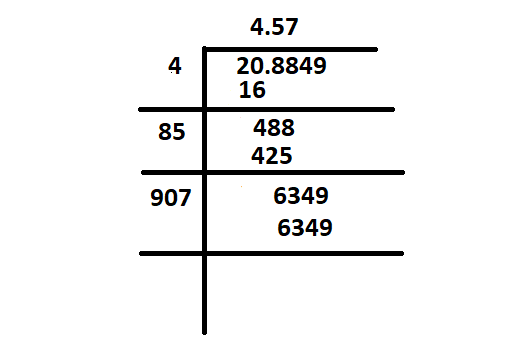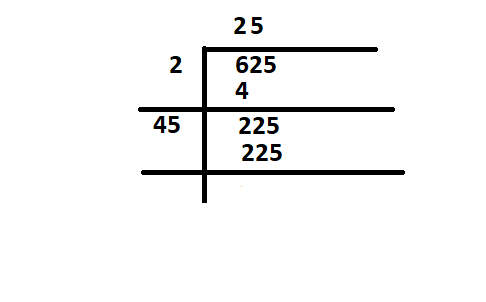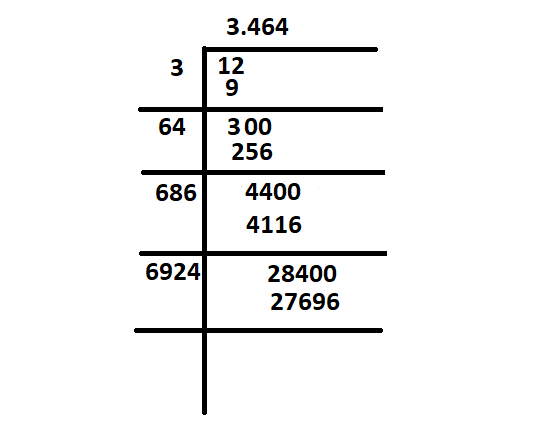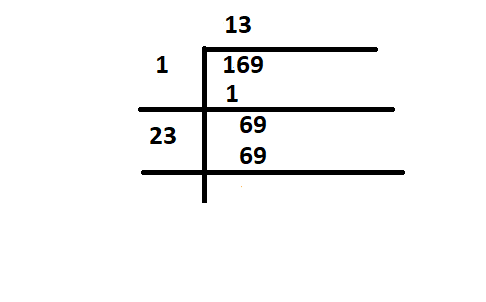How to simplify square roots?

• Last Updated : 05 Oct, 2021

The arithmetic value which is used for representing the quantity and used in making calculations are defined as Numbers. A symbol like “4, 5, 6” which represents a number is known as a numeral. Without numbers, we can’t do counting of things, date, time, money, etc., these numbers are also used for measurement and used for labeling.

The properties of numbers make them helpful in performing arithmetic operations on them. These numbers can be written in numeric forms and also in words. For example, 3 is written as three in words, 35 is written as thirty-five in words, etc. Students can write the numbers from 1 to 100 in words to learn more.

There are different types of numbers, which can be learned. They are whole and natural numbers, odd and even numbers, rational and irrational numbers, etc.

Number System

A Number System is a method of showing numbers by writing, which is a mathematical way of representing the numbers of a given set, by using the numbers or symbols in a mathematical manner. The writing system for denoting numbers using digits or symbols in a logical manner is defined as Number System.

The digits can be used from 0 to 9 to form all the numbers. With these digits, anyone can create infinite numbers. For example, 156, 3907, 3456, 1298, 784859, etc.

Square Root

The value of a number of square roots, which on multiplication by itself gives the original number. Suppose, a is the square root of b, then it is represented as a = √b or can be expressed the same equation as a2 = b. Here, ’√’ this symbol we used to represent the root of numbers is termed as radical. The positive number when it is to be multiplied by itself represents the square of the number. The square root of the square of any positive number gives the original number.

For example, the square of 4 is 16, 42 = 16, and the square root of 16, √16 = 4. Since 4 is a perfect square, hence it is easy to find the square root of such numbers, but for an imperfect square, it’s really tricky.

Square Root is represented as ‘√’. It is called a radical symbol. To represent a number ‘a’ as a square root using this symbol can be written as: ‘√a‘, where a is the number. The number here under the radical symbol is called the radicand. For example, the square root of 4 is also represented as a radical of 4. Both represent the same value.

The formula to find the square root is,

a = √b

Properties of Square Roots

It is defined as a one-to-one function that takes a positive number as an input and returns the square root of the given input number. For example, here if x = 9, then the function returns the output value as 3. In a generalized way, it can be written as,

f(x) = √x

The properties of the square root are as follows,

• If a number is a perfect square number, then they’re definitely exists a perfect square root.
• If a number ends with an even number of zeros (0’s), then we can have a square root.
• The two square root values can be multiplied. For example, √3 can be multiplied by √2, then the result will be √6.
• When two same square roots are multiplied, then the result must be a radical number. It shows that the result is a non-square root number. For example, when √7 is multiplied by √7, the result obtained is 7.
• The square root of negative numbers is undefined. Hence the perfect square cannot be negative.
• Some of the numbers end with 2, 3, 7, or 8 (in the unit digit), then the perfect square root does not exist.
• Some of the numbers end with 1, 4, 5, 6, or 9 in the unit digit, then the number will have a square root.

Note It is easy to find the square root of a number that is a perfect square.

Perfect squares

Perfect squares are those positive numbers that can be written as the multiplication of a number by itself, or you can say that a perfect square is a number which is the value of power 2 of any integer. The number can be expressed as the product of two equal integers. For example, 16 is a perfect square because it is the product of two equal integers, 4 × 4 = 16. However, 24 is not a perfect square because it cannot be expressed as the product of two equal integers. (8 × 3 = 24).

The number which is obtained by squaring a whole number is termed as a Perfect square.

Assume N is a perfect square of a whole number y, this can be written as N = the product of y and y = y2.

So, the perfect square formula can be expressed as,

N = Y2

Let’s Use the formula with values,

If y = 9, and N = y2

This means, N = 92 = 81.

Here, 81 is a perfect square of 9 because it is the square of a whole number.

With the help of square roots, one can identify whether a number is a perfect square or not, if one calculates the square root of the given number. If the square root is a whole number then the given number will be a perfect square, and if the square root value is not a whole number, then the given number is not a perfect square.

For instance, to check whether 24 is a perfect square or not, we will calculate its square root. √24 = 4.898979. As we can see, 4.898979 is not a whole number, so, 24 is not a perfect square. Let’s take another example,

There is a number 49. √49 = 7. We can see that 7 is a whole number, therefore, 49 is a perfect square.

How to simplify square roots?

The value of a number of square roots, which on multiplication by itself gives the original number. Suppose, a is the square root of b, then it is represented as a = √b or we can express the same equation as a2 = b. Here, ’√’ this symbol we used to represent the root of numbers is termed as radical. The positive number when it is to be multiplied by itself represents the square of the number. The square root of the square of any positive number gives the original number.

For example, the square of 4 is 16, 42 = 16, and the square root of 16, √16 = 4. Since 4 is a perfect square, hence it is easy to find the square root of such numbers, but for an imperfect square, it’s really tricky.

For example to simplify square roots, Square root of 24 by long division methodHere square root of 24 is 4.57    i.e √20.8849 = 4.57

Sample Problems

Question 1: Simplify the square root of 625 using the long division method?

Solution:

By normal learning of some basic square roots, students can tell that the square root of 625 is 25. However, here the demand is to solve it through the long division method.the square root of 625

Here square root of 625 is 25 i.e √625 = 25

Question 2: Simplify square root of 12 using the long division method?

Solution:

12 is not a perfect square of any number and therefore, it is now remembered properly. However, the square root of 12 can be easily taken out through the long division method,The square root of 12

Here square root of 12 is 3.464 i.e √12 = 3.464

Question 3: Simplify the square root of 169 using long division method?

Solution:

13 is not a perfect square of any number and therefore, it is now remembered properly. However, the square root of 13 can be easily taken out through the long division method,The square root of 169

Here square root of 169 is 13 , which is perfect square

My Personal Notes arrow_drop_up